Author: Oscar Cronquist Article last updated on May 11, 2022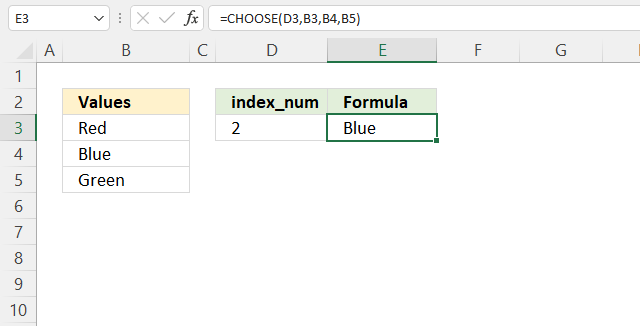The CHOOSE function lets you get a value based on a number, the number determines which value to get. The first value is 1 and the second value is 2 and so on.

Formula in cell E3:

=CHOOSE(D3, B3, B4, B5)

The CHOOSE function demonstrated above uses the specified number in cell D3 to get a value from cells B3, B4, and B5. Number two returns the value in cell B4. Cell B4 is the second cell reference.

Note, you can't use a cell range containing multiple values. You need to type each cell separated by a comma. There is an exception to this demonstrated later in this article.

## 1. CHOOSE function Syntax

CHOOSE(index_num, value1, [value2], ...)

## 2. Arguments

 Argument Text Index_num Determines which value is chosen. This argument is required, you can use a number between 1 and 254. value1, value2, value3 Up to 254 values Index_num can pick from. The first value is required the remaining values are optional.

## 3. CHOOSE function and multiple columns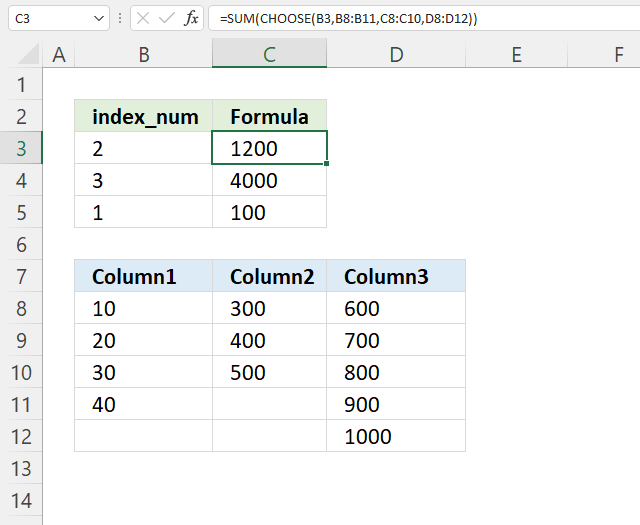The picture above displays a formula that allows you to select a cell range and then a SUM function adds all numbers in the selected cell range.

Formula in cell C3 selects cell range C8:C10 and adds the numbers 300 + 400 + 500 = 1200 is shown in cell C3.

Array formula in cell C3:

=SUM(CHOOSE(B3, B8:B11, C8:C11, D8:D11))

### 3.1 How to enter an array formula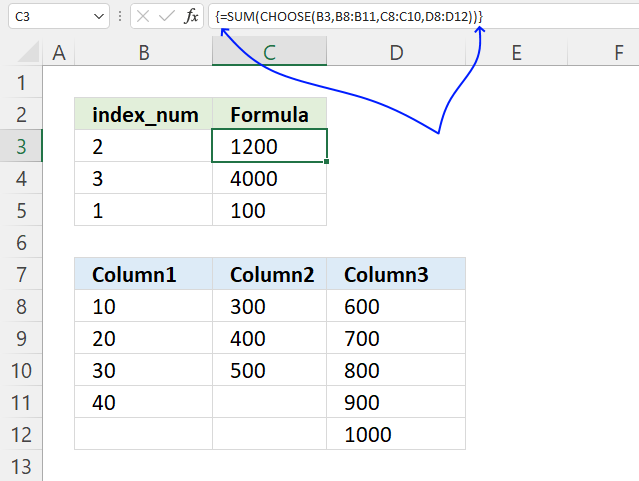1. Copy the array formula above.
2. Double press with the left mouse button on cell C3, a prompt appears.
3. Paste it to cell C3, shortcut keys are CTRL + v.
4. Press and hold CTRL + SHIFT keys simultaneously.
5. Press Enter once.
6. Release all keys.

The formula bar shows a beginning and ending curly bracket, don't enter these characters yourself.

They appear automatically if you followed the above steps.

Note, Excel 365 users can ignore these steps. Array formulas work like regular formulas in Excel 365.

### 3.2 Explaining formula

#### Step 1 - Choose cell reference

CHOOSE(B3, B8:B11, C8:C11, D8:D11)

becomes

CHOOSE(2, B8:B11, C8:C11, D8:D11)

becomes

C8:C10

and returns {300; 400; 500}. Cell reference C8:C10 is the second cell reference.

#### Step 2 - Add numbers and return a total

The SUM function calculates a sum based on a given set of numbers. It works fine with arrays as well.

SUM(number1, [number2], ...)

SUM(CHOOSE(B3, B8:B11, C8:C11, D8:D11))

becomes

SUM({300; 400; 500})

and returns 1200 in cell C3.

## 4. How to hardcode values in the CHOOSE function

Use function key F9 to quickly create hard-coded values from a specific cell range, then use the values in the CHOOSE function.

1. Double-press with left mouse button on an empty cell.
2. Type = (equal sign)
3. Select a cell range with your mouse or type a cell range.
4. Press F9 to convert values in cell range to "constants" or hard-coded values.
5. Delete the curly brackets { }.
6. Replace semicolons with colons.
7. Use the values in your CHOOSE function.

## 5. CHOOSE function array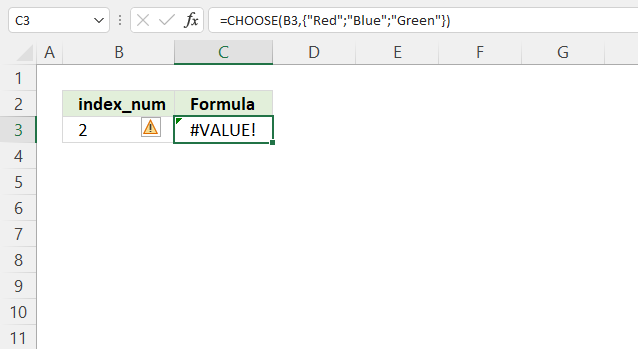The CHOOSE function can't work with arrays or cell ranges, it returns a #VALUE error if you try.

Formula in cell C3:

=CHOOSE(B3,{"Red";"Blue";"Green"})

What can you do about it? Use the INDEX function, see the example below.

## 6. CHOOSE function alternative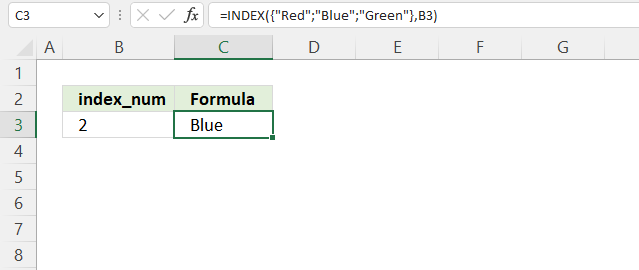Formula in cell C3:

=INDEX({"Red";"Blue";"Green"},B3)

The INDEX function has the following arguments:

INDEX(array, [row_num], [col_num], [area_num])

### Explaining formula in cell C3

INDEX({"Red";"Blue";"Green"},B3)

becomes

INDEX({"Red";"Blue";"Green"},2)

and returns "Blue" in cell C3. "Blue" is the second value in the array.

## 7. CHOOSE function drop-down list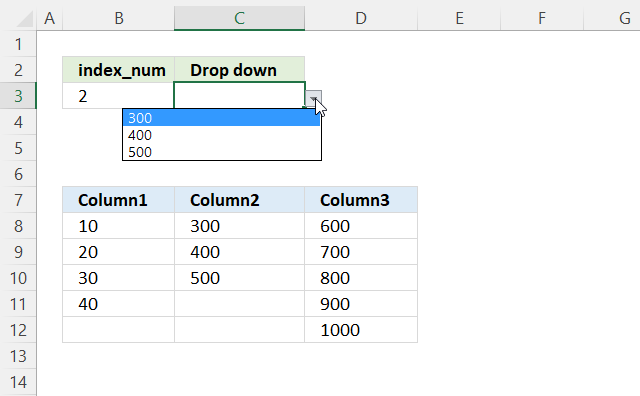The image above shows a drop-down list in cell C4, it is populated with values from a given column in B8:D12 based on the number specified in cell B3.

For example, cell B3 contains 2. The drop-down list is populated with values from the second column. Change the number to 3 in cell B3 and the drop-down list is instantly and automatically populated with values from column 3.

Drop-down list formula:

=CHOOSE(B3,B8:B11,C8:C10,D8:D12)

### 7.1 How to insert a drop-down list

1. Go to tab "Data" on the ribbon.
2. Press with the left mouse button on button "Data Validation", a dialog box appears.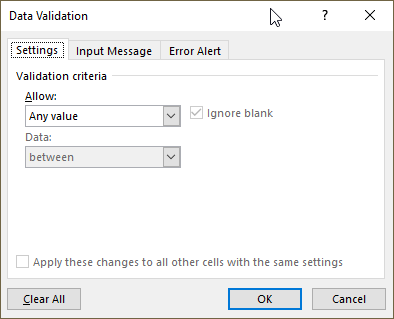3. Press with the left mouse button on drop-down list "below "Allow:" and change it to "List".
4. Press with left mouse button on in the field below "Source" and type the following formula:
=CHOOSE(B3,B8:B11,C8:C10,D8:D12)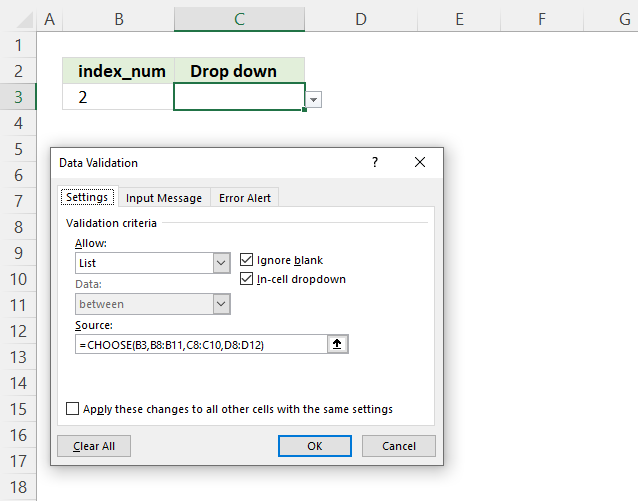5. Press with the left mouse button on "OK" button.## 8. CHOOSE function with VLOOKUP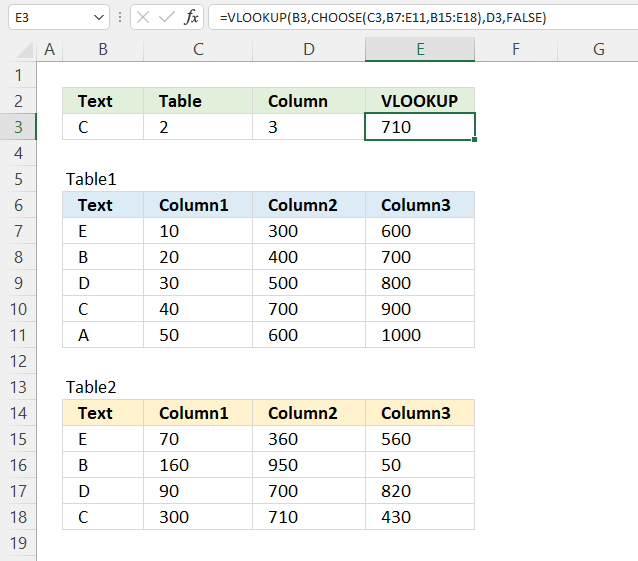This picture above demonstrates a VLOOKUP function combined with the CHOOSE function, the CHOOSE function lets you use two different data sets in B7:E11 and B15:E18. Cell C3 contains a number that determines which data set to be used.

Formula in cell E3:

=VLOOKUP(B3, CHOOSE(C3, B7:E11, B15:E18), D3, FALSE)

### 8.1 Explaining formula

#### Step 1 - Choose cell reference

CHOOSE(C3, B7:E11, B15:E18)

becomes

CHOOSE(2, B7:E11, B15:E18)

and returns B15:E18.

#### Step 2 - Find value in the chosen data set

The VLOOKUP function lets you search the leftmost column for a value and return another value on the same row in a column you specify.

VLOOKUP(lookup_value, table_array, col_index_num, [range_lookup])

VLOOKUP(B3, CHOOSE(C3, B7:E11, B15:E18), D3, FALSE)

becomes

VLOOKUP(B3, B15:E18, D3, FALSE)

becomes

VLOOKUP("C", B15:E18, 3, FALSE)

and returns the value in D18. "C" is found on row 18 in the leftmost column in B15:E18. The third argument col_index_num is 3. The intersection of row 18 and the third column is D18.

## 9. CHOOSE function return array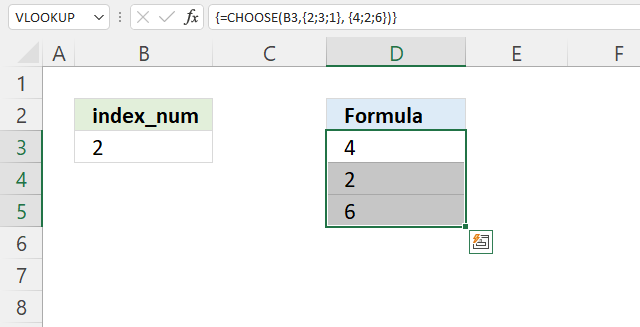The image above demonstrates how to return an array of values using the CHOOSE function.

Array formula in cell D3:

=CHOOSE(B3, {2;3;1}, {4;2;6})

Excel 365 users can enter this formula as a regular formula, previous Excel versions need to enter this as an array formula.

How to enter an array formula

### 9.1 Explaining formula

CHOOSE(B3,{2;3;1}, {4;2;6})

becomes

CHOOSE(2,{2;3;1}, {4;2;6})

and returns {4;2;6}. The CHOOSE function returns the second array.

This array has a semicolon as a delimiting character, this means that the values are vertically arranged in the array. This is why you get an array of values in column D, see the image above.

## 10. CHOOSE function from list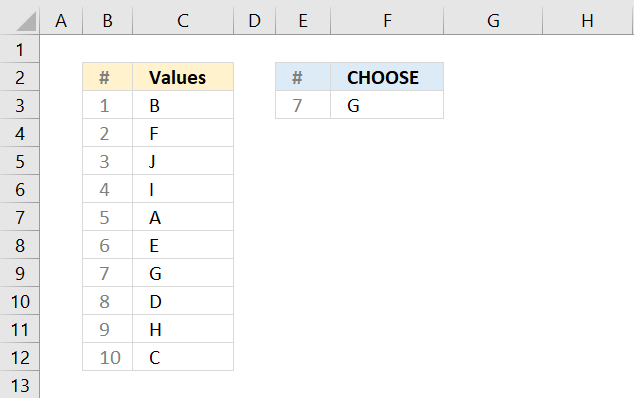The picture above shows the CHOOSE function in cell F3, one disadvantage is that you need to press with left mouse button on each cell in the list to build the formula.

=CHOOSE(E3,C3,C4,C5,C6,C7,C8,C9,C10,C11,C12)

If you have a long list that will take some time, however, there is a workaround.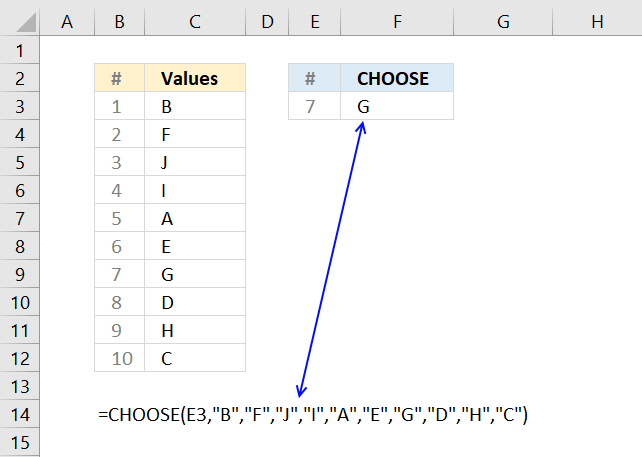You can quickly convert a cell range to hard-coded values, here are the steps.

1. Double press with left mouse button on a cell
2. Type =CHOOSE(E3,TRANSPOSE(C3:C12))
3. Select TRANSPOSE(C3:C12) in the formula with your mouse
4. Press function key F9 to convert the cell reference to values
5. Delete the curly brackets { }
6. Press Enter
=CHOOSE(E3,"B","F","J","I","A","E","G","D","H","C")

Note, you don't need the TRANSPOSE function if your values are arranged horizontally.

However, the CHOOSE function allows you to have up to 254 arguments, and perhaps hardcoded values are not what you want. If you have more than 254 values you need another solution, demonstrated below.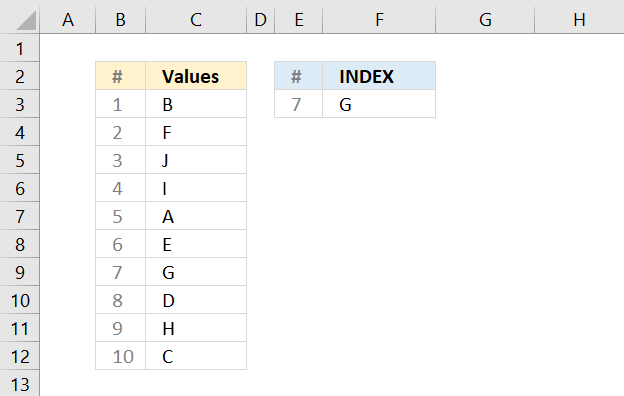The INDEX function allows you to choose a value from a cell range without the need to select each value while building the formula.

=INDEX(C3:C12,E3)